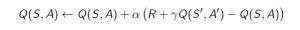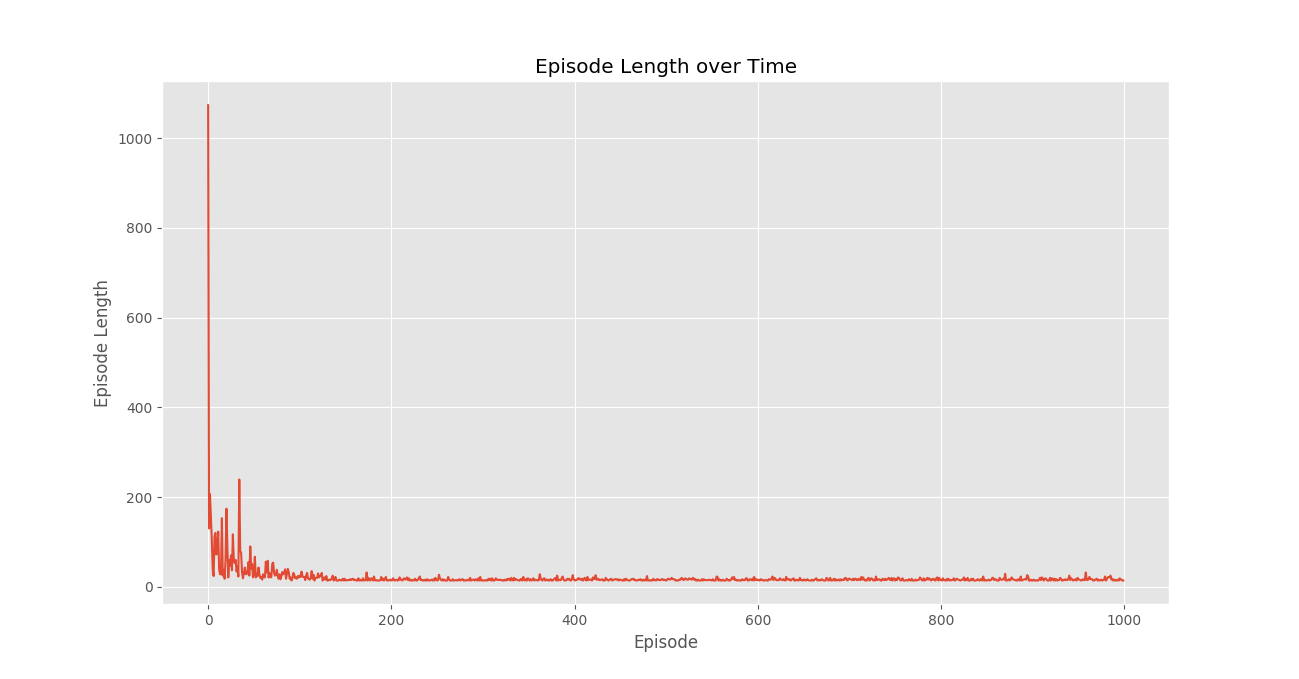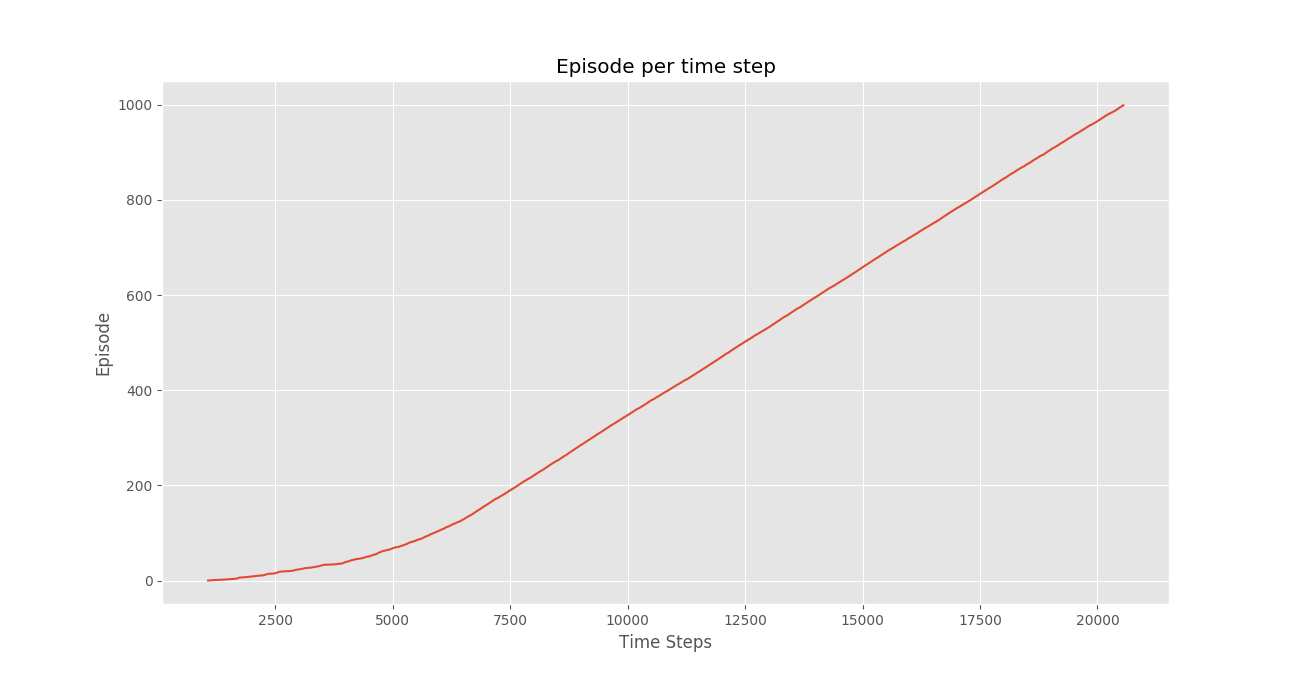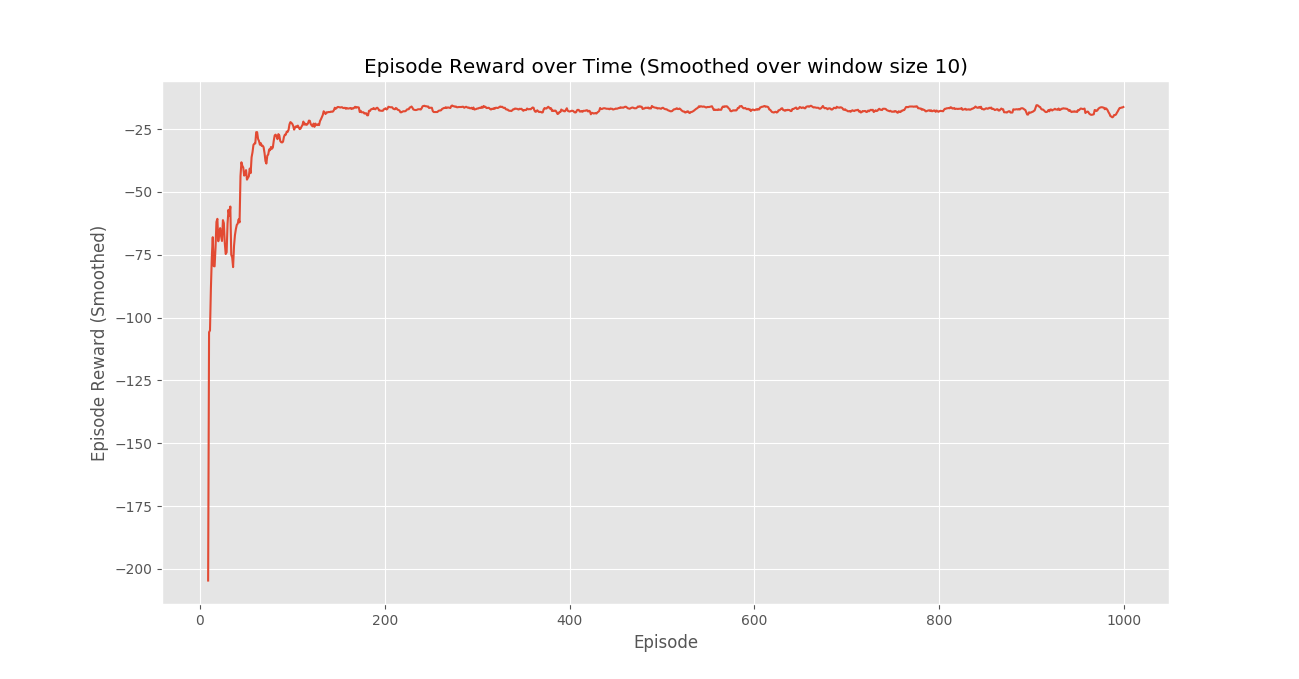# Q-Learning in Python

## Q-Learning in Python

Reinforcement Learning briefly is a paradigm of Learning Process in which a learning agent learns, overtime, to behave optimally in a certain environment by interacting continuously in the environment. The agent during its course of learning experience various different situations in the environment it is in. These are called states. The agent while being in that state may choose from a set of allowable actions which may fetch different rewards(or penalties). The learning agent overtime learns to maximize these rewards so as to behave optimally at any given state it is in.

Q-Learning is a basic form of Reinforcement Learning which uses Q-values (also called action values) to iteratively improve the behavior of the learning agent.

1. Q-Values or Action-Values: Q-values are defined for states and actions.is an estimation of how good is it to take the actionat the state. This estimation ofwill be iteratively computed using the TD- Update rule which we will see in the upcoming sections.
2. Rewards and Episodes: An agent over the course of its lifetime starts from a start state, makes a number of transitions from its current state to a next state based on its choice of action and also the environment the agent is interacting in. At every step of transition, the agent from a state takes an action, observes a reward from the environment, and then transits to another state. If at any point of time the agent ends up in one of the terminating states that means there are no further transition possible. This is said to be the completion of an episode.
3. Temporal Difference or TD-Update:The Temporal Difference or TD-Update rule can be represented as follows :This update rule to estimate the value of Q is applied at every time step of the agents interaction with the environment. The terms used are explained below. :

•: Current State of the agent.
•: Current Action Picked according to some policy.
•: Next State where the agent ends up.
•: Next best action to be picked using current Q-value estimation, i.e. pick the action with the maximum Q-value in the next state.
•: Current Reward observed from the environment in Response of current action.
•(>0 and <=1) : Discounting Factor for Future Rewards. Future rewars are less valuable than current rewards so they must be discounted. Since Q-value is an estimation of expected rewards from a state, discounting rule applies here as well.
•: Step length taken to update the estimation of Q(S, A).
4. Choosing the Action to take using-greedy policy:-greedy policy of is a very simple policy of choosing actions using the current Q-value estimations. It goes as follows :
• With probabilitychoose the action which has the highest Q-value.
• With probabilitychoose any action at random.

Now with all the theory required in hand let us take an example. We will use OpenAI’s gym environment to train our Q-Learning model.

Command to Install gym

pip install gym

Before starting with example, you will need some helper code in order to visualize the working of the algorithms. There will be two helper files which need to be downloaded in the working directory. One can find the files here.

Step # 1 : Import required libraries.

 import gym import itertools import matplotlib import matplotlib.style import numpy as np import pandas as pd import sys     from collections import defaultdict from windy_gridworld import WindyGridworldEnv import plotting   matplotlib.style.use('ggplot')

Step #2 : Create gym environment.

 env = WindyGridworldEnv()

Step #3 : Make the-greedy policy.

 def createEpsilonGreedyPolicy(Q, epsilon, num_actions):     """     Creates an epsilon-greedy policy based     on a given Q-function and epsilon.            Returns a function that takes the state     as an input and returns the probabilities     for each action in the form of a numpy array      of length of the action space(set of possible actions).     """     def policyFunction(state):            Action_probabilities = np.ones(num_actions,                 dtype = float) * epsilon / num_actions                           best_action = np.argmax(Q[state])         Action_probabilities[best_action] += (1.0 - epsilon)         return Action_probabilities        return policyFunction

Step #4 : Build Q-Learning Model.

 def qLearning(env, num_episodes, discount_factor = 1.0,                             alpha = 0.6, epsilon = 0.1):     """     Q-Learning algorithm: Off-policy TD control.     Finds the optimal greedy policy while improving     following an epsilon-greedy policy"""            # Action value function     # A nested dictionary that maps     # state -> (action -> action-value).     Q = defaultdict(lambda: np.zeros(env.action_space.n))        # Keeps track of useful statistics     stats = plotting.EpisodeStats(         episode_lengths = np.zeros(num_episodes),         episode_rewards = np.zeros(num_episodes))                # Create an epsilon greedy policy function     # appropriately for environment action space     policy = createEpsilonGreedyPolicy(Q, epsilon, env.action_space.n)            # For every episode     for ith_episode in range(num_episodes):                    # Reset the environment and pick the first action         state = env.reset()                    for t in itertools.count():                            # get probabilities of all actions from current state             action_probabilities = policy(state)                # choose action according to              # the probability distribution             action = np.random.choice(np.arange(                       len(action_probabilities)),                        p = action_probabilities)                # take action and get reward, transit to next state             next_state, reward, done, _ = env.step(action)                # Update statistics             stats.episode_rewards[ith_episode] += reward             stats.episode_lengths[ith_episode] = t                            # TD Update             best_next_action = np.argmax(Q[next_state])                 td_target = reward + discount_factor * Q[next_state][best_next_action]             td_delta = td_target - Q[state][action]             Q[state][action] += alpha * td_delta                # done is True if episode terminated                if done:                 break                                state = next_state            return Q, stats

Step #5 : Train the model.

 Q, stats = qLearning(env, 1000)

Step #6 : Plot important statistics.

 plotting.plot_episode_stats(stats)Conclusion:
We see that in the Episode Reward over time plot that the episode rewards progressively increase over time and ultimately levels out at a high reward per episode value which indicates that the agent has learnt to maximize its total reward earned in an episode by behaving optimally at every state.

Last Updated on March 1, 2022 by admin

## User-defined Exceptions in Python with ExamplesUser-defined Exceptions in Python with Examples

User-defined Exceptions in Python with Examples Python throws errors and exceptions when there is a

## What is a clean, Pythonic way to have multiple constructors in Python?What is a clean, Pythonic way to have multiple constructors in Python?

What is a clean, Pythonic way to have multiple constructors in Python? Python does not

## Python Program to convert List of Integer to List of StringPython Program to convert List of Integer to List of String

Python Program to convert List of Integer to List of String Given a List of

## Django FormsDjango Forms

Django Forms When one creates a Form class, the most important part is defining the fields of

## Logic Gates in PythonLogic Gates in Python

Logic Gates in Python Logic gates are elementary building blocks for any digital circuits. It

## Intersection() function PythonIntersection() function Python

Intersection() function Python Python intersection() function return a new set with an element that is common

## Python – How to search for a string in text files?Python – How to search for a string in text files?

Python – How to search for a string in text files? In this article, we

## Pandas dataframe.interpolate()Pandas dataframe.interpolate()

Python | Pandas dataframe.interpolate() Python is a great language for doing data analysis, primarily because